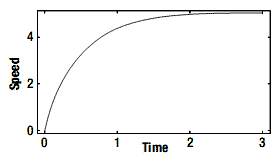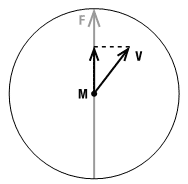# 4.4 使用加速度控制速度

## 问题## 工作原理

Vector3 modelPosition = new Vector3();
float modelYRot = 0;
Vector3 modelVelocity= new Vector3(); 

const float modelMaxAcceleration = 30.0f;
const float modelMaxTurnSpeed = 0.002f; 

float elapsedSeconds = (float)gameTime.ElapsedGameTime.Milliseconds / 1000.0f;
float forwardReq= 0;
float angleReq = 0;
if (keyState.IsKeyDown(Keys.Up)) forwardReq += 1.0f;
if(keyState.IsKeyDown(Keys.Down)) forwardReq -= 1.0f;
if (keyState.IsKeyDown(Keys.Left))angleReq += 1.0f;
if (keyState.IsKeyDown(Keys.Right)) angleReq -= 1.0f; 

### 在光滑表面上加速

Matrix rotMatrix = Matrix.CreateRotationY(angle);
Vector3 forwardDir = Vector3.Transform(new Vector3(0, 0, -1), rotMatrix);
velocity = velocity + elapsedTime * forwardReq *maxAccel *forwardDir;
modelPosition += velocity;
modelYRot += rotationReq * maxRotSpeed* velocity.Length(); 

### 添加摩擦力

velocity = velocity * (1 - friction * elapsedTime) + elapsedTime * forwardReq *maxAccel *forwardDir;

### 让模型保持在向前方向运动float forwardSpeed = Vector3.Dot(velocity, forwardDir);
velocity = forwardSpeed * forwardDir;
modelPosition += velocity * elapsedTime;
modelYRot+= rotationReq * maxRotSpeed * forwardSpeed; 

forwardSpeed变量表示Velocity矢量在Forward方向上分量的大小，然后乘以Forward方向并将结果存储在一个新的Velocity矢量中。通过这个方法，你可以保证模型将沿着向前方向移动。

private float Accelerate(ref Vector3 position, ref float angle, ref Vector3 velocity,float forwardReq,
float rotationReq, float elapsedTime, float maxAccel, float maxRotSpeed,float friction)
{
Matrix rotMatrix = Matrix.CreateRotationY(angle);
Vector3 forwardDir= Vector3.Transform(new Vector3(0, 0, -1), rotMatrix);

velocity = velocity * (1- friction * elapsedTime) + elapsedTime * forwardReq * maxAccel *forwardDir;
loat forwardSpeed = Vector3.Dot(velocity, forwardDir);
velocity = forwardSpeed * forwardDir;

modelPosition += velocity * elapsedTime;
modelYRot += rotationReq * maxRotSpeed* forwardSpeed;

return forwardSpeed;
}

## 扩展阅读

2006 - 2023，推荐分辨率1024*768以上，推荐浏览器Chrome、Edge等现代浏览器，截止2021年12月5日的访问次数：1872万9823 站长邮箱